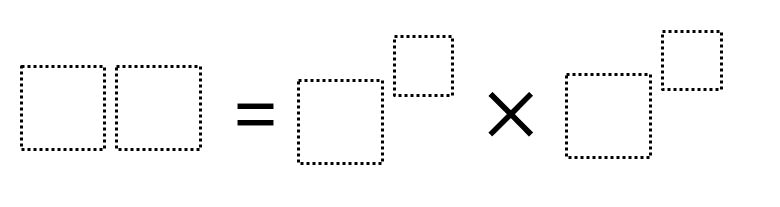Home > Grade 6 > Prime Factorization 2

# Prime Factorization 2

Directions: Using the digits 0 to 9 at most one time each, fill in the boxes to make the greatest possible product.### Hint

What digits would make good/bad exponents?
What digits would make good/bad bases?

The greatest product that’s been found so far is 80 = 5^1 * 2^4.

Source: Robert Kaplinsky

## Fraction of an Amount

Directions: Using the digits from 1 to 9, at most once, make as many true …

1.2.we got 81= 9^2 x 7^0

•81 multiply by zero is zero

•But anything (except 0)to the power of 0 is 1, not 0.

•3.i did _____________=9^8×7^6

•80=5^1×2^4

•I 96 because 6^1 × 4^2 is equal to that.

4.i did 96=2^5+3^1

5.6.Why not 96=2^5+3^1

7.8.Danitza Marenco

That´s exactly what i thought 96=2^5+3^1

9.I think it would be 80 = 5^1*2^4

•5.0644e12 =

10.5.0644e12 =

11.96=2^5 x 3^1

12.80=5^1 * 2^4

13.14.You did a terrific job! This post seem extremely great.

15.I am not very sure but i am guessing that it would be 93= 2^5* 3^2 This is kinda confusing to me so i think i might be a bit off

16.I did 9^2*3*2=

17.I got 96 = 6^1 x 4^2

•You used the digit 6 twice.

18.I did 64=1^0*8^2 because anything to the power of one equals zero.